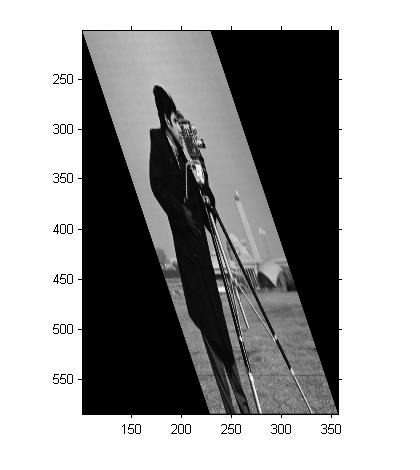# Viewing output-space coordinates for a transformed image

Blog reader Ram asked a question last week that I hear fairly often: When you apply a spatial transformation to an image, how can you see the x-y coordinates when you display the image?

1. Ask imtransform for the spatial coordinates of the output image

2. Give imshow the spatial coordinates when you display the output image

3. Turn on the axis box and tick labels

Let's walk through these steps using an affine transformation.

T = maketform('affine',[.5 0 0; .5 1.5 0; 100 200 1]);
I = imread('cameraman.tif');

The first output from imtransform is the transformed image. There are two more optional arguments, though: xdata and ydata. xdata is a two-element vector specifying the x-coordinates of the first and last image columns. Similarly, ydata specifies the y-coordinates of the first and last image rows.

[I2, xdata, ydata] = imtransform(I, T);
xdata
xdata =

101   356


ydata
ydata =

201.5000  584.5000



Step 2 is to pass the coordinate information to imshow. You can do that by using the 'XData' and 'YData' parameters, like this:

imshow(I2, 'XData', xdata, 'YData', ydata)But we still can't see the coordinates! That's because imshow turns the axis display off. That brings us to step 3: Turn on the axis box and tick labels:

axis onAfter you do these steps, you might also want to call impixelinfo, which turns on a display of pixel location and value in the figure window. The display updates as you move the mouse.

Published with MATLAB® 7.6

|

### コメント

コメントを残すには、ここ をクリックして MathWorks アカウントにサインインするか新しい MathWorks アカウントを作成します。